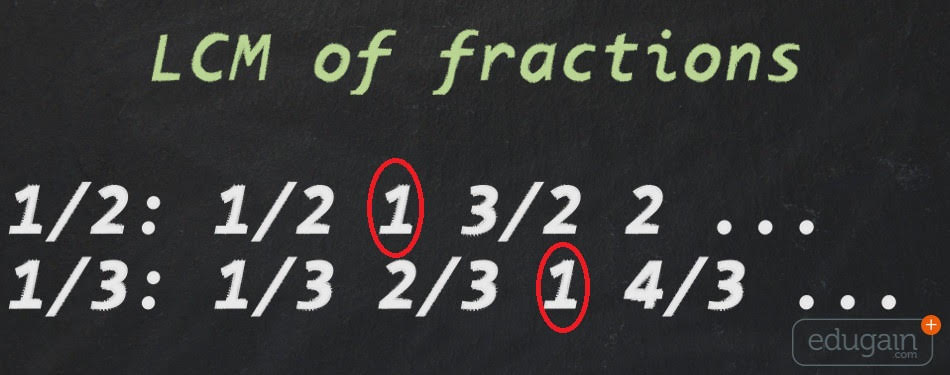### LCM of Fractions## LCM of Fractions

The Least Common Multiple (LCM) of two integers x and y, is the smallest positive integer that is a multiple of both x and y. Generally LCM is used for adding fractions where denominators are not same. Most of the kids know how to calculate LCM, but I was surprised to learn that most of the kids are not aware of the physical significance of LCM.

I was interacting with some of the grade 6 students and I asked them about LCM, and all kids in class said that they know LCM very well. So I asked them to find LCM of 1/2 and 1/3 and surprisingly no one could answer. They were trying to apply regular method of finding LCM of integers, and of-course that did not help them in finding LCM of fractions.

Problem was that kids just learn the method of solving questions in textbooks, and do not pay much attention to theory. Had any student used the definition of LCM (and not the regular method of finding LCM) they could have easily solved this question.

Anyway lets forget the regular method of finding LCM and try to solve this using definition of LCM.

So as per the definition of LCM, we have to find a number which can be fully divided by 1/2 and 1/3. If you think about it you will find that answer is 1. Since one is fully divisible by both 1/2 and 1/3.

1/ (1/2) = 2
1/ (1/3) = 3

Now lets take another example. Find LCM of 1/6 and 1/9.
Answer for this one is 1/3, since,
(1/3) / (1/6) = 2
(1/3) / (1/9) = 3

Ok, these were simple cases so we could do just by thinking about it, but we might have to do this for more complex fractions. Now lets formalize a method to find LCM of any two fractions.

Lets try to find LCM of (a/b) and (c/d). If b and d were same it was easy to find LCM since if denominators are same, we just need to find LCM of numerators, hence LCM of (a/b) and (c/b) would be LCM(a,c)/b. So we have to first make denominators of both the fractions same.

So here are the steps to find LCM of a/b and c/d

1. Find the LCM of b and d = LCM(b,d)
2. Multiply numerator and denominator of first fraction by LCM(b,d)/b.
Multiply numerator and denominator of first fraction by LCM(b,d)/d.
After this multiplication, denominator of both fractions are same.
3. Find LCM of new numerators.

Lets see this using example of 2/9 and 8/21.

1. LCM of 9 and 21 = 63,
2. Now multiply first fraction by 63/9 = 7
Multiply second fraction by 63/21 = 3
So now first fractions is (2 x 7)/(9 x 7) = 14/63
and second fraction is (8 x 3)/(21 x 3) = 24/63
3. Now since both denominators are same, LCM of numerators 14 and 24 = 168.
4. Hence LCM of 2/9 and 6/21 is (168/63)
After simplification 168/63 = 8/3

Now lets check if our answer (8/3) is correct or not by dividing this by 2/9 and 8/21.
(8/3) / (2/9) = 12
(8/3) / (8/21) = 7

You can see that this is fully divisible by both fractions.

Now we have understood the concept, lets try to find a formula for quickly solving this.
If you observe the new numerators after multiplication, they are (a/b)*LCM(b,d) and (c/d)*LCM(b,d).